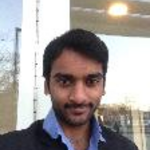Community Profile# Nikhil Prathapani

### University of Maryland, College Park

Active since 2013

Highly contributive in the fields of Telecommunications, Image Processing and Nanoelectronics
Professional Interests: Telecommunications, Nano electronics,Image Processing

#### Statistics

•••#### Content Feed

View by

Submitted

Sampling Theorem Illustration
Sampling is basically coversion of continuos time signals to discrete time signalsSubmitted

Amplitude Modulation and DSBSC wave generation
Amplitude modulation (AM) is a modulation technique used in electronic communication.Submitted

Frequency Modulation (FM)
FM is the encoding of information in a carrier by varying the instantaneous frequency of the waveSubmitted

Time scaing for continuous signals
To perform Time scaing on continuous time signalsSubmitted

Time scaling for discrete signals
To perform Time scaling on discrete time signalsSubmitted

Time folding for sequences
Performing Time folding operation on sequencesSubmitted

Time folding for continuous signals
Performing Time folding operation on continuous signalsSubmitted

Addition and Multiplication of Discrete time signals
Performing Addition and Multiplication of Discrete time signalsSubmitted

Continuous Sinc and Discrete Sinc
Generation of Sinc signal in continuous and discrete time.Submitted

Continuous Triangular wave and Discrete Triangular wave
Generation of continuous and discrete triangular waveSubmitted

Continuous gate and Discrete gate
Generation of Gate(Rectangular) wave in continuous and discrete time.Submitted

Continuous sawtooth and Discrete sawtooth
Generation of sawtooth wave in continuous and discrete time.Submitted

Continuous Cosine and Discrete Cosine
Generation of Cosine signal in continuous and discrete time.Submitted

Continuous sine and Discrete SIne
Generation of Sine signal in continuous and discrete time.Submitted

Continuous ramp and Discrete ramp
Generation of Ramp signal in continuous and discrete time.Submitted

Addition and Multiplication of Continuous time signals
Performing addition and multiplication of continuous time signalsSubmitted

Continuous square and Discrete square
Generation of Square wave in continuous and discrete time.Submitted

Continuous Unit Step and Discrete Unit Step
Generation of Unit Step signal in continuous and discrete time.